# Rubik’s Cube Superflips and God’s Number

• How hard is it to solve a Rubik's Cube?
• When can you say that your Rubik's Cube is completely scrambled?
• Why might the answer depend on where you went to school?
• What interesting mathematical questions are involved?

### Contents

#### Metrics

The difficulty of solving any configuration of a Rubik's Cube is the smallest number of moves required to return to the intial configuration where each face is showing a single color.

But what, exactly, is a move? The so-called quarter-turn metric says a move is turning any face by 90 degrees. The half-turn metric says turning any face by either 90 or 180 degrees is a single move. For example, using Singmaster notation and the quarter-turn metric, the sequence "L L", which turns the left face twice in the same direction, is two moves. But in the half-move metric, the sequence becomes "L2" and counts as a single move.

Tomas Rokicki, who describes himself as a programmer from Palo Alto, provides some history at cube20.org.

In the early days of cube mathematics, two camps emerged on how to measure the difficulty of a position. West coast and Stanford mathematicians, free thinkers all, tended to prefer the half-turn metric, where any twist of any face, whether 90 degrees, 180 degrees, or 270 degrees counted as a single move. The east coast crowd, including MIT, tended to prefer the rigor of the quarter-turn metric, where a half-turn counted as two moves, since of course it could be accomplished by two consecutive quarter turns.

When I began development of a Rubik's Cube simulator, Qube, I was unaware of this history and, even though I am a devout West-coaster, I just counted quarter-turns. Now a toggle switch in Qube allows use of either metric.

#### God's number

Let Q denote a cube position,

| Q | = minimum number of moves to solve Q,

Q = the set of all possible Q's, and

G(Q) = maximum over Q in Q of | Q |.

G(Q) is known as "God's number". Q contains over 4.3*10^19 positions, so computing G(Q) is a formidable optimization problem. The definition of God's number does not require the optimal solution itself, only the number of moves.

#### Superflip

The superflip of Rubik's cube is a configuration where the 8 corners, the 6 face centers, and the cube center show the initial colors, but the 12 edge cubelets have the colors reversed. In 1995, Michael Reid proved that solution of the superflip requires 20 half-turn metric moves. In 2010, Tomas Rokicki and colleagues, using hundreds of computers at Google, carried out a massive computation to prove that no other configuration took more than 20 moves, cube20.org. This established that God's number for the half-turn metric is

G(Q) = 20

#### Q20

I use Q20 to denote the superflip. Our first animation generates the superflip with 20 moves. A few rotations at the beginning and at the end are shown in more detail so that we can see the rotation matrices involved. A higher resolution video clip is available at this link: Q20.mp4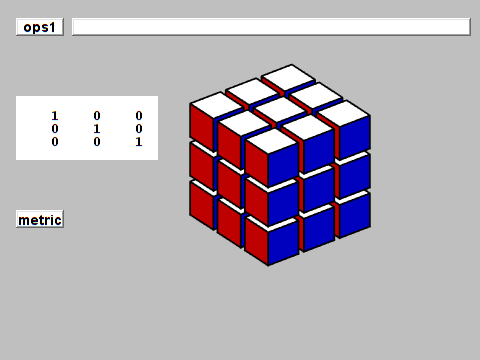The second animation shows a solution of Q20 in 20 moves obtained by reversing and complementing the generating moves. Reid's proof shows that any other solution requires at least 20 moves. The high resolution clip is: Q20solve.mp4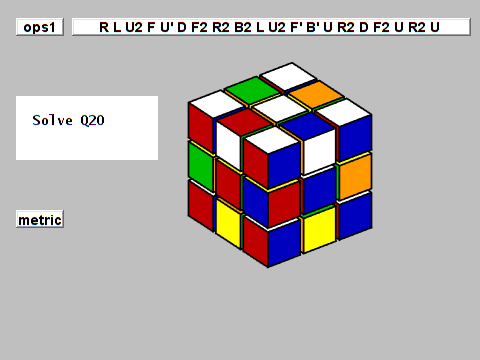There are several other configurations that require 20 moves. Any configuration with G(Q) = 20 can be regarded as completely shuffled in the half-turn metric.

#### Q26

For the quarter-turn metric, if you combine superflip and a configuration known as fourspot you have Q26 . Only 8 corners and two face enters are correct. The edges, four face centers, and the cube center are all reversed. When 180 degree turns are counted as two 90 degree turns, this configuration is generated by 26 moves and solved by reversing and complementing the 26 moves. The high resolution clip is: Q26.mp4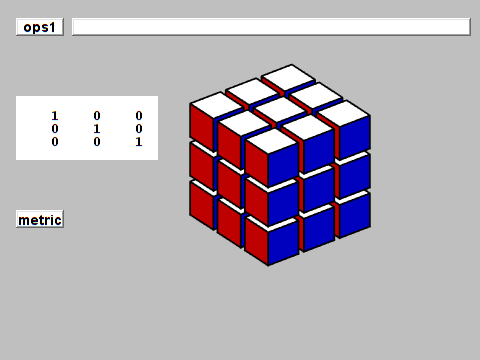In 2014, a massive computation at the Ohio Supercomputer Center by Rokicki and Morley Davidson proved that only Q26 (and its two rotations) required 26 quarter-turn moves All other configurations need fewer. cube20.org. So, this established that God's number for the half-turn metric is

G(Q) = 26

The high resolution clip is: Q26solve.mp4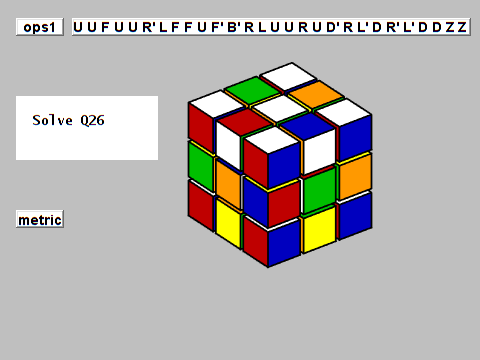#### Compare

Let's compare Q20 and Q26 by alternating between the two.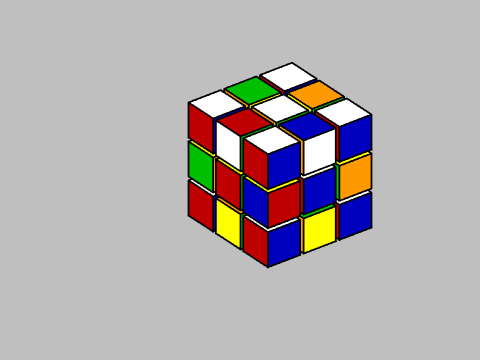The type 3 corner pieces are the same in Q20 and Q26, and are in the correct initial position.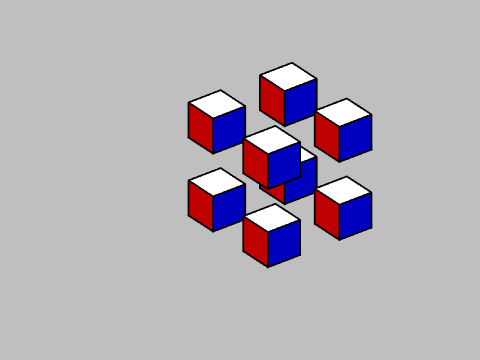The type 2 edge pieces are also the same in Q20 and Q26, but are reversed from their initial position.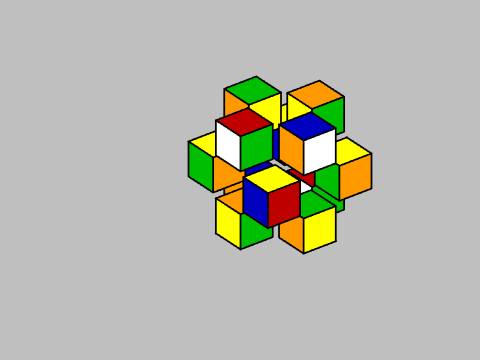All the action is with cubelets of type 0 and type 1. In a real, physical Rubik's Cube, this is one solid piece that holds the Cube together.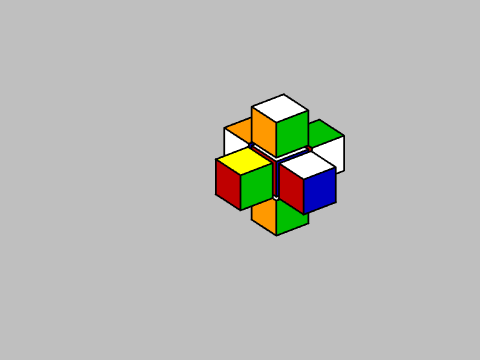Published with MATLAB® R2022b

|

### 댓글

댓글을 남기려면 링크 를 클릭하여 MathWorks 계정에 로그인하거나 계정을 새로 만드십시오.